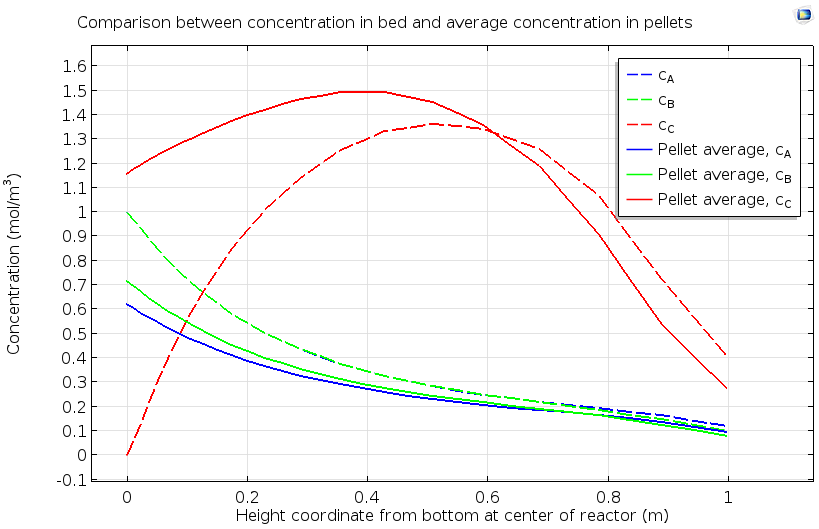# 模拟多孔介质和活性颗粒床中的表面反应

2017年 10月 10日

### 多孔催化剂和均质模型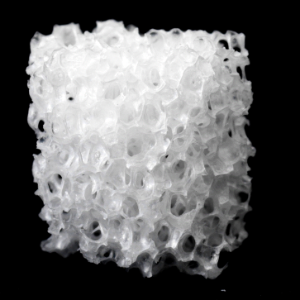• 孔隙率：空隙（流体）体积与总体积的比率
• 渗透性：流速与流动驱动力的比率，用于量化多孔介质中的表面施加的阻力
• 曲折率：实际扩散长度与点对点位移的比率，量化由非线性扩散路径引起的较低有效扩散率

R_m = \mathrm{SSA} \times N_m

R_m = k_{\mathrm{eff},m} c

k_{\mathrm{eff},m} = k_m \times \mathrm{SSA}

### 理想的方形通道反应器：多孔介质属性示例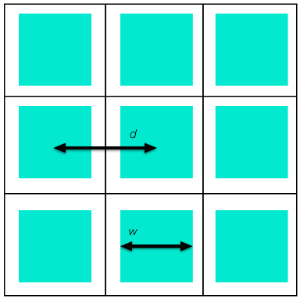\epsilon = \frac{\mathrm{pore\:volume}}{\mathrm{total\:volume}} = \frac{w^2L} {d^2L} = \left(\frac{w}{d} \right)^2

\mathrm{SSA} = \frac{\mathrm{pore\:surface\:area}}{\mathrm{total\:volume}} = \frac{4wL}{d^2L} = \frac{4\epsilon} {w}

\frac{\mu U} {\nabla p} = \left(\frac{w^2}{32}\right)

\kappa = \epsilon \frac{w^2} {32} = \frac{\epsilon^3}{2(\mathrm{SSA})^2}

t_\mathrm{diff} = \frac{r_\mathrm{eff}^2}{8D} = \frac{w^2}{8\pi D}

r_\mathrm{diff} \ll r_\mathrm{conv}

\frac{w^2} {8\pi D} \ll \frac{L}{U}

### 催化转化器中的多孔介质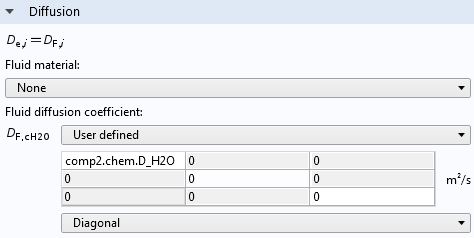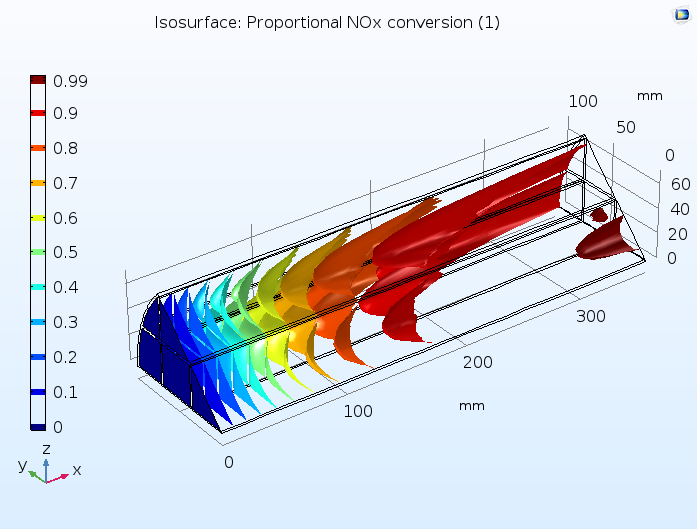### 跨尺度均相反应：反应颗粒床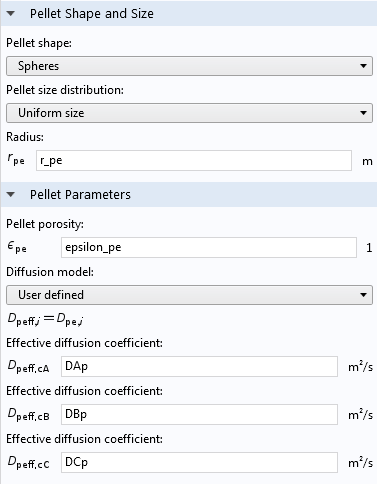• A(aq) 从流动入口到多孔填充床的对流主导传递
• 填充床孔隙中的 A(aq) 向催化剂颗粒表面的扩散主导传递
• A（aq）沿微孔的扩散
• A（aq）在微孔表面反应生成B（aq）
• B（aq）沿微孔扩散回催化剂表面
• B(aq) 从催化剂颗粒表面到流过填充床孔隙的溶液的扩散主导传递
• B(aq) 从多孔填充床本体到流动出口的对流主导传递